Standard Solutions for Volumetric Analysis

Key Concepts

• A standard solution is a solution of accurately known concentration.
• A standard solution can be prepared by dissolving a primary standard in a suitable solvent (such as distilled water).
• A primary standard is a soluble solid compound that is very pure, with a consistent formula that does not change on exposure to the atmosphere, and has a relatively high molar mass.
• A secondary standard is a solution of known concentration derived from a primary standard.

No ads = no money for us = no free stuff for you!

Characteristics of a Primary Standard

A primary standard is prepared from solid compounds.

In order to be suitable for use as a primary standard, a compound must:

• be readily available (and inexpensive)
• be very pure (analytical reagent grade, 99.9% pure)
• have a known formula (eg, degree of hydration must not change)
• be unaltered in air during weighing, that is, the compound must NOT
(a) absorb moisture from the air
(b) absorb carbon dioxide from the air
(c) be oxidised by air
• have a relatively large molar mass (to minimise errors during weighing)
• be soluble (in the solvent used, and under the conditions used, to make the solution)

Primary Standards for Acid-Base Titrations

Most alkali hydroxides, such as sodium hydroxide, NaOH, are NOT suitable for use as a primary standard because they

• readily absorb moisture, H2O, from the atmosphere
• readily absorb carbon dioxide, CO2, from the atmosphere

Hydrochloric acid, HCl, and sulfuric acid, H2SO4, are NOT suitable for use as a primary standard because although they are both commercially available as concentrated solutions that are easily diluted, the concentration of the "concentrated" solution is NOT accurately known.
Nitric acid, HNO3, is NOT suitable for use as a primary standard because it always contains a little nitrous acid, HNO2, which has a destructive action on many acid-base indicators.

Some compounds that are suitable as primary standards for acid-base titrations are:

• anhydrous sodium carbonate, Na2CO3, a weak base
• potassium hydrogen phthalate, KH(C8H4O4), a weak acid

Primary Standards for Redox Titrations

Potassium permanganate, KMnO4, is NOT suitable for use as a primary standard because it

• cannot be obtained pure enough
• readily reacts with traces of organic material or other reducing substances in water
• decomposes in sunlight

Potassium dichromate, K2Cr2O7, is suitable for use as a primary standard.

Do you know this?

Play the game now!

Preparation of a Primary Standard

Preparing Anhydrous Sodium Carbonate

Anhydrous sodium carbonate can be used as a primary standard. Anhydrous sodium carbonate is sodium carbonate that does NOT contain water of crystallisation.
Sodium carbonate is available commercially as an analytical reagent, 99.9% purity, which contains a little water.
Therefore, before the solid sodium carbonate can be used, the water must be removed by heating.

 Step 1. Weight out approximately 2 grams of solid sodium carbonate on a clean, dry watch glass.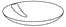watch glass Step 2. Place watchglass in an oven at a temperature above 100oC (to ensure all the water is evoparated out) but less than 270oC (to ensure the carbonate does not decompose). A temperature of approximately 260oC is often used. Step 3. After 30 minutes, remove the watchglass from the oven and transfer it immediately to a dessicator to prevent the sodium carbonate from re-absorbing water from the atmosphere.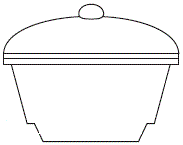dessicator Step 4. Allow the sodium carbonate to cool in the dessicator before re-weighing it. Step 5. Repeat this heating-cooling-weighing process until a constant weight is achieved (indicating that all the water has been driven off).

Preparation of a Primary Standard Solution of Sodium Carbonate

This section describes the procedure for preparing 250.00 mL of 0.04906 mol L-1 standard solution of sodium carbonate.

 Step 1. Place a small amount of distilled water into a clean, dry, 250.00 mL volumetric flask. Swirl the water around the whole interior of the flask to rinse it. Discard the water. If the water leaves the flask with a thin layer of water covering the surface of the flask, then the flask is clean. If the water is present as droplets inside the flask, the flask is dirty. It will need to be washed with detergent, rinsed well, and dried before it can be used.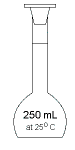volumetricflask Step 2. Weigh out 1.300 g of the anhydrous sodium carbonate prepared above onto a clean dry watchglass or small beaker. You need to work quickly because sodium carbonate is hygroscopic, it absorbs moisture from the atmosphere. Step 3. Position a clean, dry glass funnel into the neck of the volumetric flask. Leave a small air gap around the stem of the funnel inside the neck of the volumetric flask.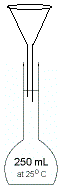funnel placed in volumetric flask with air gap Step 4. Place some of the sodium carbonate into the funnel, and wash it into the volumetric flask with a little distilled water. Repeat this process until all the sodium carbonate has been transferred to the volumetric flask. Finally, position the watchglass or beaker over the funnel and run a little distilled water over this to transfer any microscopic amounts of sodium carbonate still adhering to the watchglass or beaker to the volumetric flask. Repeat this process. Swirl the volumetric flask gently to dissolve the sodium carbonate. Do not shake the flask. Do not invert the flask. Just swirl gently. Step 5. Pour distilled water through the funnel slowly until the water level in the volumetric flask reaches the base of the neck. Allow any water still remaining in the funnel to run through (there shouldn't be any if you maintained the air gap, and, if you poured slowly.) Carefully pour distilled water through the funnel very slowly until the bottom of the meniscus is about 1 cm from the graduation mark on the neck of the volumetric flask. Step 6. Remove the funnel from the volumetric flask.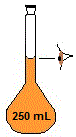reading from bottom of mensicus Step 7. Use a pasteur pipette to transfer distilled water drop by drop to the volumetric flask until the bottom of the meniscus sits on the graduation mark on the neck of the volumetric flask when viewed at eye level. Step 8. Stoppper the volumetric flask and invert it several times to ensure thorough mixing. Step 9. Label your volumetric flask with the name (and/or formula) of the reagent, its concentration and the date it was prepared. (It is a good idea to also put your name on the label so you know which solution is yours later!) [Na2CO3(aq)]=0.04906 M date 1/1/2000 (Chris the Chemist)

Do you understand this?

Take the test now!

Calculating Concentration of a Primary Standard

You will know:

• the name, and formula, of the compound you used as a primary standard
• m, the mass of this compound that you weighed out and transferred to volumetric flask
• V, the volume of the volumetric flask that you used to make the primary standard solution
(Note that this volume is probably in milliltres, mL)

You will need to calculate:

• M, the molar mass of the compound used as the primary standard
M(compound) = sum of the molar mass of each element multiplied by the number of atoms present in the formula
• n, the amount in moles of the compound you used as the primary standard
n = m ÷ M
• V, the volume of solution in litres
convert mL to L: V(L) = V(mL) ÷ 1000
• c, the concentration in mol L-1 of the primary standard solution you prepared
c = n ÷ V

For the preparation of the sodium carbonate primary standard solution above:

• formula: Na2CO3
• M(Na2CO3) = (2 x 22.99) + 12.01 + (3 x 16.00) = 105.99 g mol-1
• m(Na2CO3(s)) = 1.300 g
• n(Na2CO3(s)) = m ÷ M = 1.300 ÷ 105.99 = 0.012265 mol
• V(Na2CO3(aq)) = 250.00 mL
convert volume in mL to volume in L: 250.00 mL ÷ 1000 mL/L = 0.25000 L
• c(Na2CO3) = n ÷ V = 0.012265 ÷ 0.25000 = 0.04906 mol L-1

Concentration of the sodium carbonate primary standard solution prepared above is 0.04906 mol L-1.

Can you do this?

Join AUS-e-TUTE!

Do the drill now!

Secondary Standard Solutions

As noted above, substances like hydrochloric acid and sulfuric acid are not suitable for use as primary standards because the concentration of the commercially available concentrated acids is not known accurately.
However, you can prepare a dilute solution of these acids with an approximate concentration, and then determine the concentration accurately by titration with a primary standard solution of a base such as sodium carbonate.
Because the accurate concentration of acid has been derived using a primary standard, the acid is referred to as a secondary standard.

Similarly, alkali hydroxides such as sodium hydroxide are unsuitable for use as primary standards because they absorb moisture and carbon dioxide from the atmosphere.
However, you can prepare a sodium hydroxide solution with an approximate concentration by weighing out some NaOH pellets and dissolving them in distilled water in a volumetric flask.
This solution can then be titrated with an acidic primary standard solution, such as potassium hydrogen phthalate, KH(C8H4O4), in order to determine the concentration of the basic solution more accurately.
Because the accurate concentration of the base has been derived using a primary standard, the base is referred to as a secondary standard.

Can you apply this?

Join AUS-e-TUTE!

Take the exam now!

Worked Examples

Question 1.

0.800 g of anhydrous sodium carbonate, Na2CO3(s), is transferred to a 150.0 mL volumetric flask and made up to the mark with distilled water.
What is the concentration of the aqueous sodium carbonate solution in mol L-1 in the flask?

Worked Solution:

1. What is the question asking you to do?
Calculate the concentration of aqueous sodium carbonate solution, Na2CO3(aq), in mol L-1.
c(Na2CO3(aq)) = ? mol L-1
2. What information (data) has been given in the question?
Name of solute: anhydrous sodium carbonate
Chemical formula of solute: Na2CO3
Name of solvent: water (distilled water)
m = mass of solute = mass Na2CO3(s) = 0.800 g
V = volume of solution = volume Na2CO3(aq) = 150.0 mL
3. What is the relationship between what you know and what you need to find out?
n = m ÷ M
n = moles of solute in mol
m = mass of solute in g
M = molar mass of solute in g mol-1 (or g/mol)

c = n ÷ V
c = concentration of solution in mol L-1 (mol/L or M)
V = volume of solution in L

4. Calculate the moles of solute, n
m(Na2CO3(s)) = 0.800 g
M(Na2CO3(s)) = (2 x 22.99) + 12.01 + (3 x 16.00) = 105.99 g mol L-1

n(Na2CO3(s)) = m(Na2CO3(s)) ÷ M(Na2CO3(s))
n(Na2CO3(s)) = 0.800 ÷ 105.99 = 0.007548 mol

5. Calculate concentration of solution, c, in mol L-1
n(Na2CO3(s)) = 0.007548 mol
V(Na2CO3(aq)) = 150.0 mL
Convert volume in millilitres to volume in litres:
V(L) = v(mL) ÷ 1000 = 150.0 ÷ 1000 = 0.1500 L

c(Na2CO3(aq)) = n(Na2CO3(s)) ÷ V(Na2CO3(aq))
c(Na2CO3(aq)) = 0.007548 ÷ 0.1500 = 0.0503 mol L-1

Question 2.

What mass of potassium hydrogen phthalate, KH(C8H4O4), in grams, must be weighed out in order to make 200.0 mL of 0.250 mol L-1 KH(C8H4O4)(aq) ?

Worked Solution:

1. What is the question asking you to do?
Calculate the mass of potassium hydrogen phthalate.
m(KH(C8H4O4)) = ? g
2. What information (data) has been given in the question?
Name of solute: potassium hydrogen phthalate
Chemical formula of solute: KH(C8H4O4)
Chemical formula of solution: KH(C8H4O4)(aq)
V = volume of solution = 200.0 mL
c = concentration of solution = 0.250 mol L-
3. What is the relationship between what you know and what you need to find out?
n = c x V
n = moles of solute in mol
c = concentration of solution in mol L-1 (mol/L or M)
V = volume of solution in L

m = n x M
m = mass of solute in g
M = molar mass of solute in g mol-1 (or g/mol)

4. Calculate moles of solute, n
c(KH(C8H4O4(aq))) = 0.250 mol L-1
V(KH(C8H4O4(aq))) = 200.0 mL
Convert volume in millilitres to volume in litres:
V(KH(C8H4O4))= V(L) = v(mL) ÷ 1000 = 200.0 ÷ 1000 = 0.2000 L

n(KH(C8H4O4)) = c(KH(C8H4O4)) x V(KH(C8H4O4)) = 0.250 x 0.2000 = 0.0500 mol

5. Calculate mass of solute, m, in grams
n(KH(C8H4O4)) = 0.0500 mol
M(KH(C8H4O4)) = 39.10 + 1.008 + (8 x 12.01) + (4 x 1.008) + (4 x 16.00) = 204.22 g mol-1

m(KH(C8H4O4)) = n(KH(C8H4O4)) x M(KH(C8H4O4))
m(KH(C8H4O4)) = 0.0500 x 204.22 = 10.2 g

Can you apply this?

Join AUS-e-TUTE!

Take the exam now!

Problem Solving

(Using the StoPGoPS approach to problem solving)

The Problem:

Bo the Biologist wants to know the carbonate content of some egg shells. So Bo takes the egg shells to Chris the Chemist. Chris has decided to react the egg shells with hydrochloric acid, HCl(aq), in order to determine the carbonate content. The hydrochloric acid in the laboratory has a concentration of about 0.2 mol L-1 but the concentration of the hydrochloric acid will need to be known accurately before it can be used in the analytical procedure. Chris has decided to standardise the hydrochloric acid by titrating it with a standard solution of sodium carbonate. The balanced chemical equation for this reaction is shown below:

2HCl(aq) + Na2CO3(aq) → 2NaCl(aq) + CO2(g) + H2O(l)

Each mole of Na2CO3 requires two moles of HCl for the neutralisation reaction, so Chris has decided to prepare a standard solution of sodium carbonate that is half the concentration of the hydrochloric acid. In the laboratory, Chris has a 20.00 mL pipette which will be used to measure out volumes of the Na2CO3(aq), and a 50.00 mL burette which will be used to measure out the volume of HCl(aq). Because Chris will need to repeat the titration several times, 250.0 mL of standard Na2CO3(aq) will be required. Determine the mass, in grams, of anhydrous sodium carbonate that Chris will need to use to prepare the standard solution.

Solving the Problem

Using the StoPGoPS model for problem solving:

 STOP! State the question. What is the question asking you to do? Determine the mass, in grams, of anhydrous sodium carbonate m(anhydrous sodium carbonate) = m(Na2CO3(s)) = ? g PAUSE! Plan. What information (data) have you been given? c(HCl(aq) approximately) ≈ 0.2 mol L-1 Balanced chemical equation: 2HCl(aq) + Na2CO3(aq) → 2NaCl(aq) + CO2(g) + H2O(l) Stoichiometric (mole) ratio: HCl:Na2CO3 is 2:1 V(pipette) = V(Na2CO3 aliquot) = 20.00 mL V(burette for HCl) = 50.00 mL c(Na2CO3(aq)) = half the concentration of HCl(aq) V(standard solution) = V(Na2CO3(aq)) = 250.0 mL What is the relationship between what you know and what you need to find out? Step 1. Calculate the concentration of standard sodium carbonate solution, Na2CO3(aq) in mol L-1 Assume the concentration of HCl(aq) is 0.2 mol L-1 c(Na2CO3(aq)) = half the concentration of HCl(aq) c(Na2CO3(aq)) = ½ x c(HCl(aq)) mol L-1 Step 2. Calculate the moles of Na2CO3 in Na2CO3(aq) Assume the only source of sodium carbonate is the anhydrous sodium carbonate used to make the solution, that is, there is no sodium carbonate in the water used to make the solution. Assume the conditions used to make the solution are the same as those required by the volumetric glassware (the temperature at which the glassware is calibrated is usually printed on volumetric glassware) n(Na2CO3) = moles(Na2CO3) mol V(Na2CO3(aq)) in litres = V(Na2CO3(aq)) in mL ÷ 1000 mL/L n(Na2CO3) = c(Na2CO3(aq)) x V(Na2CO3(aq)) Step 3. Calculate mass of Na2CO3(s) Assume the solid anhydrous sodium carbonate to be used is 100% pure, that is, no impurities. m(Na2CO3(s)) = mass(Na2CO3(s)) in grams M(Na2CO3) = molar mass of Na2CO3 in g mol-1 (use Periodic Table) m(Na2CO3(s)) = n(Na2CO3) x M(Na2CO3(s)) GO! Go. Go with the game plan! Step 1. Calculate the concentration of standard sodium carbonate solution, Na2CO3(aq) in mol L-1 Assume the concentration of HCl(aq) is 0.2 mol L-1 c(Na2CO3(aq)) = half the concentration of HCl(aq) c(Na2CO3(aq)) = ½ x c(HCl(aq)) mol L-1 = ½ x 0.2 = 0.1 mol L-1 Step 2. Calculate the moles of Na2CO3 in Na2CO3(aq) Assume the only source of sodium carbonate is the anhydrous sodium carbonate used to make the solution, that is, there is no sodium carbonate in the water used to make the solution. Assume the conditions used to make the solution are the same as those required by the volumetric glassware (the temperature at which the glassware is calibrated is usually printed on volumetric glassware) n(Na2CO3) = moles(Na2CO3) mol V(Na2CO3(aq)) in litres = V(Na2CO3(aq)) in mL ÷ 1000 mL/L = 250.0 ÷ 1000 = 0.2500 L n(Na2CO3) = c(Na2CO3(aq)) x V(Na2CO3(aq)) = 0.1 x 0.2500 = 0.025 mol Step 3. Calculate mass of Na2CO3(s) Assume the solid anhydrous sodium carbonate to be used is 100% pure, that is, no impurities. m(Na2CO3(s)) = mass(Na2CO3(s)) in grams M(Na2CO3) = molar mass of Na2CO3 in g mol-1 (use Periodic Table) = (2 x 22.99) + 12.01 + (3 x 16.00) = 105.99 g mol-1 m(Na2CO3(s)) = n(Na2CO3) x M(Na2CO3(s)) = 0.025 x 105.99 = 2.6 = 3 g Note that there is justification for only 1 significant figure (since we have assumed that the concentration of HCl is 0.2 mol L-1). Also note that when Chris the Chemist performs the experiment, the mass of Na2CO3(s) weighed out and used will be recorded accurately. PAUSE! Ponder plausability. Does this solution answer the question that was asked? Yes, we have determined the mass, in grams, of anhydrous sodium carbonate, Na2CO3(s), required. Is the solution reasonable? The mass of anhydrous sodium carbonate, Na2CO3(s), needed was calculated to be 3 g We can work backwards to check the solution: moles Na2CO3(s) = mass/molar mass = 3/105.99 = 0.03 mol concentration Na2CO3(s) = moles/volume = 0.03 ÷ 250/1000 = 0.1 mol L-1 concentration HCl = 2 x concentration Na2CO3(s) = 2 x 0.1 = 0.2 which is the same as the concentration of HCl given in the question so we are confident our solution is plausible. STOP! State the solution. State the solution to the problem: The mass of anhydrous sodium carbonate required is 3 g

Can you apply this?

Join AUS-e-TUTE!

Take the exam now!